# Space framesThe structural frame
The construction
The reinforcement I
The reinforcement II
Quantity/Cost estimation
Detailing drawings
Introduction >Wind and Seismic Forces >
Structural model and Analysis
Slabs
Seismic behavour of frames
Appendix A
Appendix B
Appendix C
Appendix D
Introduction >
Modelling slabsMaterials
To be continued >
Introduction

## Diaphragmatic behaviour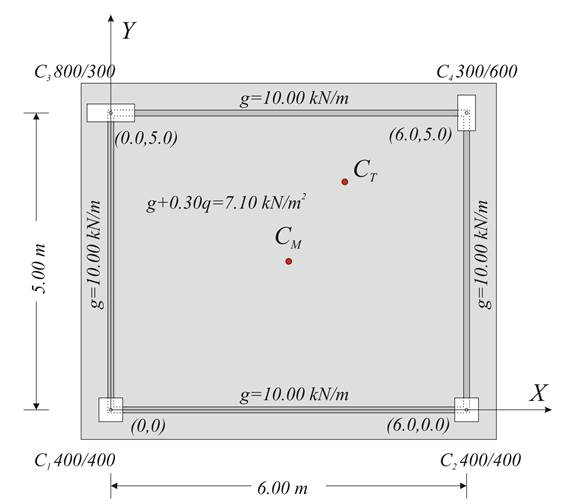Simple one-storey structure comprising four columns whose tops are connected by rigid slab-diaphragm

Floor slabs act in storey frames as strong horizontal elements, called diaphragms. Diaphragms are practically rigid and non-deformable and thus impose specific movements to the beams and the column tops.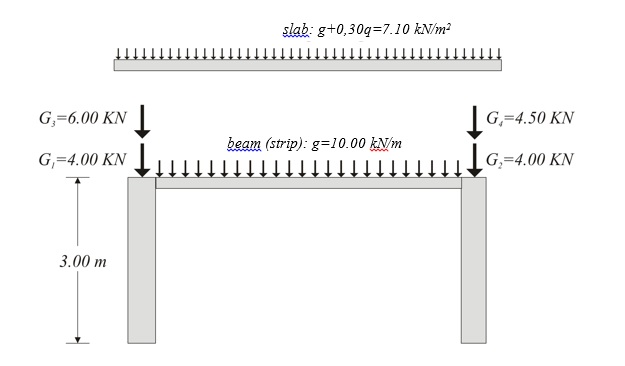In case of an earthquake event, during which the main stresses are induced by the horizontal seismic actions, diaphragm action affects directly the behaviour of the whole structure.
The diaphragmatic behaviour of structures of any type and size is presented in the following par-agraphs. The simple four-column structure of the previous page is used as an example.

## Centre of mass and radius of gyration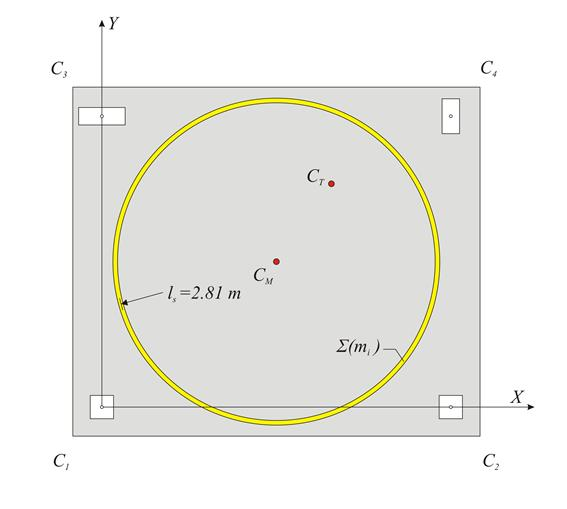The centre of mass CM and the equivalent mass inertial ring with radius of gyration ls

The inertial behaviour of a diaphragm having a mass Σ(mi) is  idealised by a ring of equivalent inertial mass distribution. The ring has a total mass Σ(mi), its centre is the Centre of Mass CM and its radius the Radius of Gyration ls.

The coordinates of the centre of mass CM of a diaphragm consisting of many mass points, linearly distributed, and surface masses linearly distributed derive from the expressions: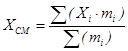,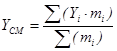(1)

The radius of gyration ls of the diaphragm with respect to the centre of mass CM derives from the expression: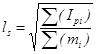(2)

where Χand Υare the coordinates of each mass point mof the diaphragm, whereas Ipi is the polar moment of inertia of each mass mi  with respect to the centre of mass CM.

## Example of calculating centre of mass and radius of gyration

Centre of mass:

Due to symmetrical mass distribution, XCM=3.0 m and YCM=2.5 m.

Radius of gyration: [g=10 m/sec2, therefore force F=1 kN corresponds to mass m=0.1 t.]

Slab: m1=6.0m·5.0m·0.71t/m2=21.3 t

b1=6.0 m, l1=5.0 m, L1=0.0 m Ip1=21.3t·(6.02+5.02)m2/12=108.3 t·m2

Beam connecting C1-C2: m2=6m·1.0t/m=6.0 t, l2=6.0 m, L2=2.5 m Ip2=6.0·(62/12+2.52)=55.5 t·m2

Beam connecting C3-C4: likewise m3=6.0 t, Ip2=55.5 t·m2

Beam connecting C1-C3: m4=5.0m·1.0t/m=5.0 t, l4=5.0 m, L4=3.0 m Ip4=5.0·(5.02/12+3.02)=55.4 t·m2

Beam connecting C2-C4: likewise m5=5.0 t, Ip5=55.4 t·m2

Columns: m6=0.1·(4.00+4.00+6.0+4.5)=1.85 t, L6=√ (3.02+2.52)=3.905 m   Ip6=1.85·3.9052=28.2 t·m2.

Finally Σ(mi)=45.3 t and Σ(Ipi)=358.3 t·m2 (2) ls=√[(Σ(Ipι)/Σ(mi)]=√(358.3/45.3)=2.81 m

## Centre of stiffness and elastic displacements of the diaphragm Subject description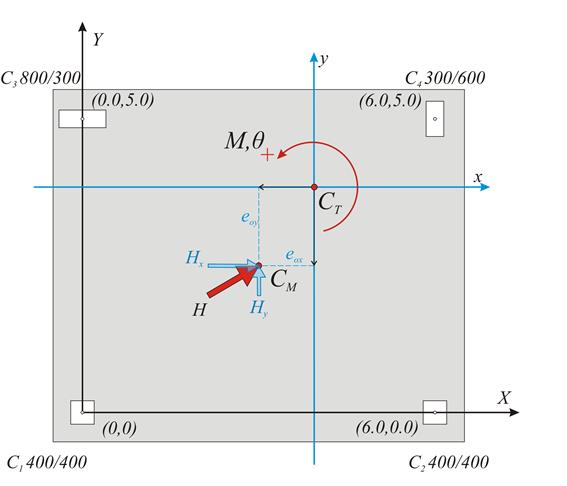Simple one-storey structure comprising four columns, whose tops are connected by a rigid slab-diaphragm

The current paragraph examines the special case of orthogonal columns in parallel arrangement. The general case is examined in Appendix C.

## Parallel translation of the diaphragm in both directions and rotation , due to a force Η applied to the centre of mass CM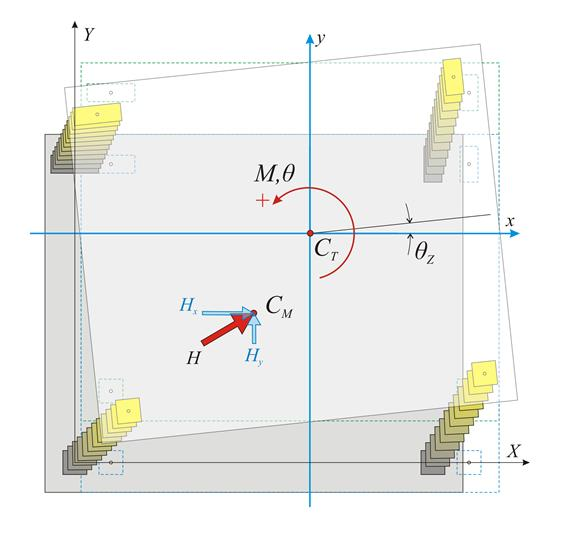Χ0Υ initial coordinate system, xCTy main coordinate system

When a horizontal force H acts on a storey level, all the points of the slab including the column tops move in accordance with the same rules due to the in-plane rigidity of the slab.

These rules induce the diaphragm to develop a parallel (translational) displacement by δxo, δyo and a rotation θz about the centre of stiffness CT(xCT, yCT) in xCTy coordinate system, which is parallel to the initial coordinate system X0Y and has as origin the point CT.

The diaphragmatic behaviour may be considered as a superposition of three cases:

(a) parallel translation of the diaphragm  along the X direction due to horizontal force component HX,

(b) parallel translation of the diaphragm  along the Y direction due to horizontal force component HY,

(c) rotation of the diaphragm due to moment MCT applied at the centre of stiffness CT.

## Translation of centre of stiffness CT along x direction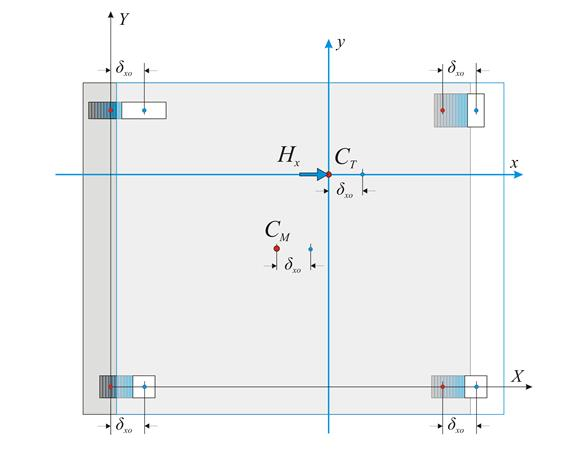In case a horizontal force Hx is applied at CT in x direction, the following 2 equilibrium equations apply:

·         The sum of forces in x direction is equal to Hx, i.e. Hx=Σ(Vxoi) (i).

·         The sum of moments Vxoi about the point CT is equal to zero, i.e. Σ(Vxoi·yi)=0 (ii).

Each column i carries a shear force Vxoi=δxo·Kxi.

Σ(Vxoi)=Σ(δxo·Kxi)=δxo·Σ(Kxi),  expression (i) gives Hx=δxo·Σ(Kxi)

Hx=Kx·δxo where Kx=Σ(Kxi).

Expression (ii) gives Σ(Vxoi·[Yi-YCT])=0 Σ(Vxoi·Yi )-Σ(Vxoi·YCT)=0 YCT·Σ(Vxoi)= Σ(Vxoi·YCT)

YCT=Σ(δxo·Kxi·Yi)/Σ(δxo·Kxi) YCT=Σ(Kxi·Yi)/Σ(Kxi)

## Translation of centre of stiffness CT along y direction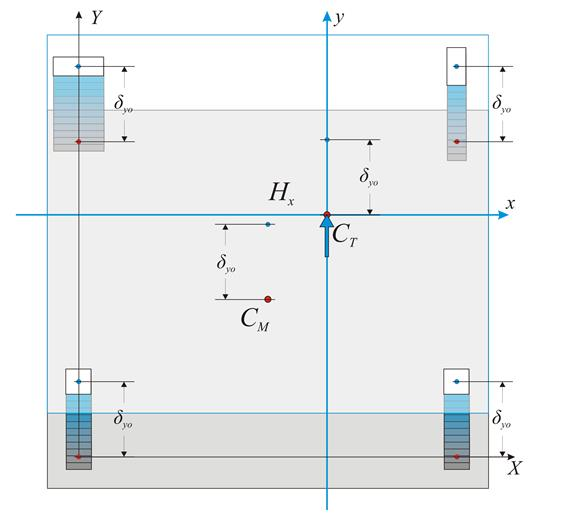Accordingly, the corresponding expressions are derived for direction y.

Hy=Ky·δyo where Ky=Σ(Kyi) and XCT=Σ(Kyi·Xi)/Σ(Kyi)

Summarising, the centre of stiffness and the lateral stiffnesses are defined by the following expressions:

Centre of stiffness and lateral stiffnesses: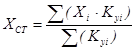,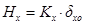where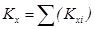(4’)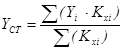,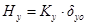where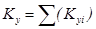(5’)

## Rotation of the diaphragm by an angle θz about CT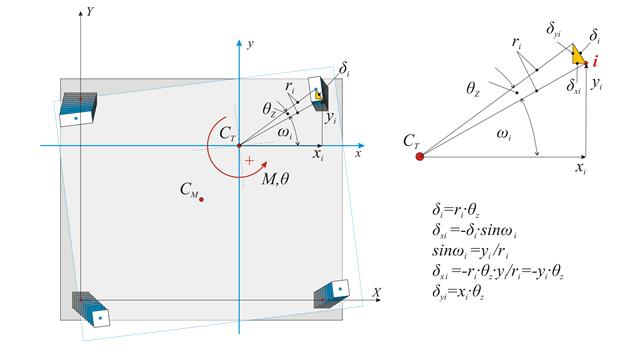To determine the deformation developed by external moment M, applied at the centre of stiffness CT, the initial system X0Y is transferred (by parallel translation) to the principal system xCTy. The centre of mass is transferred to the principal system along the structural eccentricities eox, eoy in accordance with the following expressions:

Principal coordinate system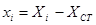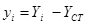,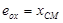,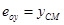(6’)

The displacement of the diaphragm consists essentially of a rotation θz about the CT, inducing a displacement δi at each column top i with coordinates xi,yi in respect to the coordinate system with origin the CT. If the distance between the point i and the CT is ri, the two components of the (infinitesimal) deformation δi are equal to δxi=-θz·yi and δyi=θz·xi.

The shear forces Vxi and Vyi in each column developed from the displacements δxi, δyi are:

Vxi=Kxi·δxi=Kxi·(-θz·yi) Vxi=-θz·Kxi·yi and Vyi=Kyi·δyi=Kyi·(θz·xi) Vyi=θz·kyi·xi

The resultant moment of all shear forces Vxi, Vyi about the centre of stiffness is equal to the external moment MCT, i.e.

MCT=Σ(-Vxi·yi+Vyi·xi+Kzi) MCT= θz·Σ(Kxi·yi2+Kyi·xi2+Kzi)

Torsional stiffness Kzi of column i

Columns resist the rotation of the diaphragm by their flexural stiffness expressed in terms Kxi·yi2 , Kyi·xi2 (in N·m), and their torsional stiffness Kzi, which is measured in units of moment e.g. N·m.

The  torsional stiffness of a column is given by the expression  Kz=0.5E·Id/h, where 0.5Ε is the material shear modulus G, usually taken equal to 0.5 of the elasticity modulus, h is the height of the column and Id is the torsional moment of inertia of the column’s cross-section, taken from the following table.

Torsional stiffness of the floor diaphragm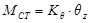, where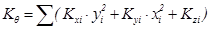(7’)

The quantity Κθ is the torsional stiffness of the diaphragm and is measured in N·m. The quantities Kx=Σ(Kxi), Ky=Σ(Kyi), measured in N/m, imply the lateral stiffnesses of the diaphragm in x and y direction respectively.

Definitions:

Lateral stiffness Κj of diaphragm denotes the force in direction j required to cause a relative parallel displacement of the diaphragm by one unit in considered direction.

Torsional stiffness Kθ of diaphragm denotes the moment required to cause relative rotation of the diaphragm by one unit.

Note

The torsional stiffness of columns Kz is very small and is usually omitted.

## Torsional moment of inertia of the column’s cross-section

 Cross-sectional shape Id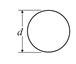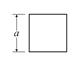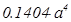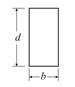b the shorter side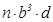where n is taken from the following expression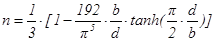## Torsional stiffness ellipse, torsional radii and equivalent system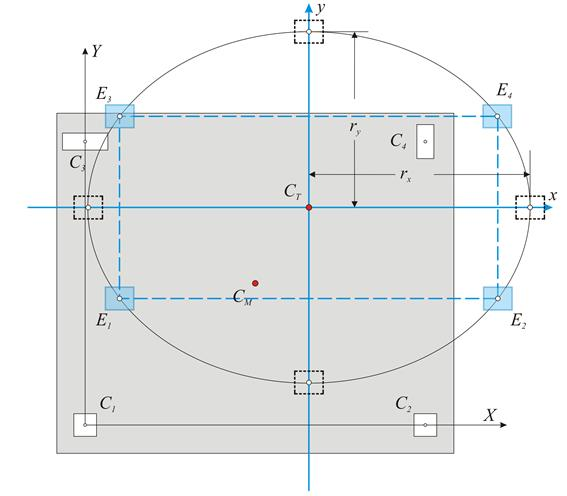Question: Create a simple idealised equivalent structural system with the same seismic behaviour as the actual structural system.

Solution: Four idealised columns E1, E2 and E3, E4 are placed symmetrically with respect to the centre CT and the axes x and y, i.e. all four idealised columns have the same absolute value of coordinates x and y. Each idealised column is assumed to have stiffnesses Kx=1/4·Σ(Kxi) and Ky=1/4·Σ(Kyi).

This system satisfies the two conditions of the actual system, concerning the translations of all diaphragm columns.

Stiffness by x: 4·1/4·Σ(Kxi)=Σ(Kxi) and stiffness by y: 4·(1/4)·Σ(Kyi)=Σ(Kyi)

To satisfy the third condition, the torsional stiffness of the idealised system should be

Kθ,eq=[4·(1/4)·Σ(Kyi)·y2+4·(1/4)·Σ(Kxi)·x2]=Σ(Kxi)·y2+Σ(Kyi)·x2

equal to the torsional stiffness of the actual system

Kθ,re=Κθ=Σ(Kxi·yi2+Kyi·xi2+Kzi).

i.e. Kθ,eq=Kθ,re Σ(Kyi)·x2+Σ(Kxi)·y2=Kθ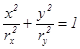where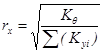and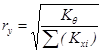(8’)

The curve equation (8’) is an ellipse with centre CT, direction that of the principal axes (in this case the direction of the initial system) and semi axes rx, ry, (torsional radii of the diaphragm).

Conclusion:

The torsional behaviour of a floor can be described by the torsional stiffness ellipse (CT, rx, ry) which represents the equivalent distribution of the diaphragm stiffness.

There are infinite solutions of idealised dual system sets, whose most characteristic is the one with the four idealised columns placed in the four ellipse ends.

Generally, there are infinite solutions with n-tuple diametrically opposed systems, where each idealised column stiffness is equal to 1/n of the total system stiffness.

## Assessment of building torsional behaviour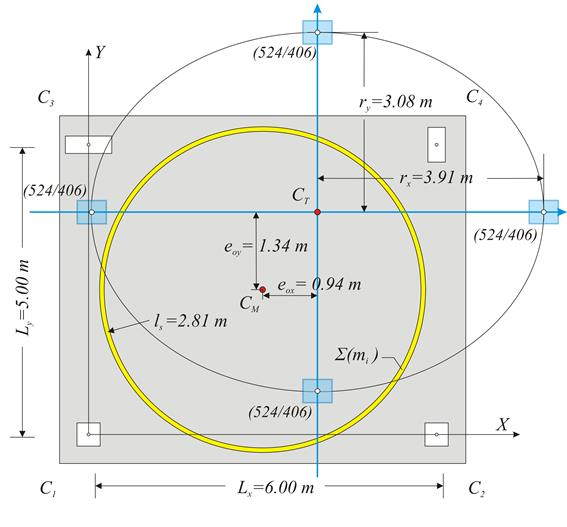Equivalent mass inertial ring (CM, ls) and torsional stiffness ellipse (CT, rx, ry)

The degree of the torsional stiffness of a diaphragmatic floor is determined by the relation between the equivalent mass inertial ring (CM, ls) and the torsional stiffness ellipse (CT, rx, ry). The optimal location of the two curves is where the torsional stiffness ellipse encloses the mass inertial ring.

A building is classified as torsionally flexible [EC8 §5.2.2.1] if either rx<ls or ry<ls is satisfied in at least one diaphragm storey level. In this example both conditions are satisfied.

For a building to be categorized as being regular in plan, the two structural eccentricities eoxeoy   at each level  shall satisfy both conditions eox≤0.30r&eoy≤0.30r [EC8 §4.2.3.2]. In this particular example the first condition is satisfiedeox=0.94 m ≤ 0.30rx (=0.30·3.91=1.173 m), whereas the second one is not eox=1.34 m ≤ 0.30rx (=0.30·3.08=0.924 m). Therefore the building that comprises that specific floor diaphragm is not regular in plan.

## One-storey space frame with rectangular columns in parallel arrangement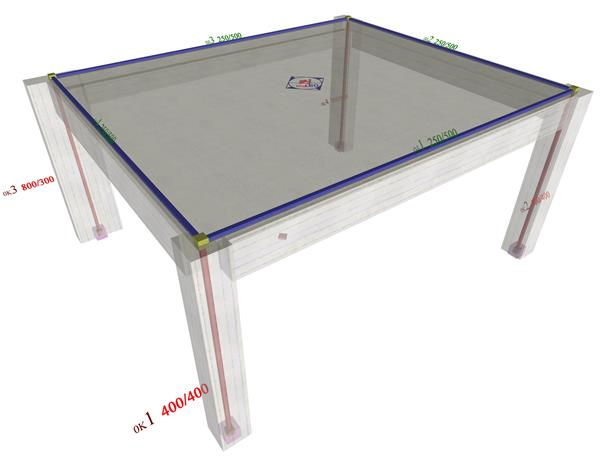The structure and the model of the one-storey space frame

The analysis of the one-storey space frame illustrated in the figure, under horizontal seismic force

H=90.6 kN

is performed by means of four methods: (i) analysis using manual calculations, assuming fixed-ended columns, (ii) analysis using the Excel file, assuming fixed-ended columns, (iii) analysis using the Excel file, assuming columns with k=6, (iv) analysis using software, assuming

actual

beam and column torsional stiffnesses.

## Analysis using the Excel file, assuming fixed-ended columns (k=12)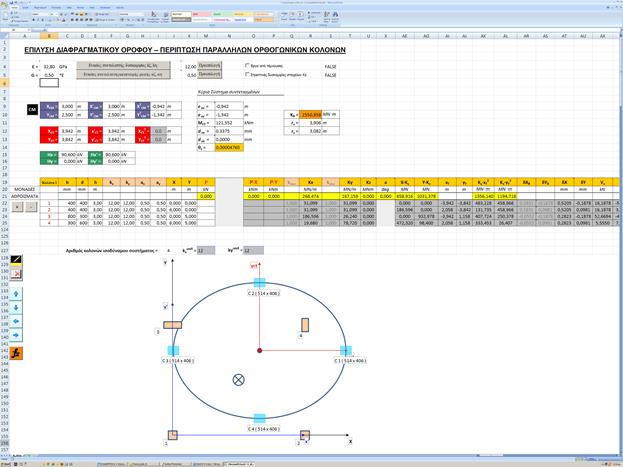The method using manual calculations, was described via the example presented to all previous paragraphs of this chapter.
The results are identical to those of the practical calculations.
The torsional stiffness ellipse and the equivalent columns of the structure are illustrated at the end of the spreadsheetς.

## Analysis using the Excel file, assuming fixed-ended columns (k=6)

The centre of stiffness, the torsional radii and the stress resultants are exactly the same as those of the previous cases, with the exception of deformations, whose relative values, however, re-main constant 12/6=2.00.

## Analysis using software, assuming actual beam and column torsional stiffnesses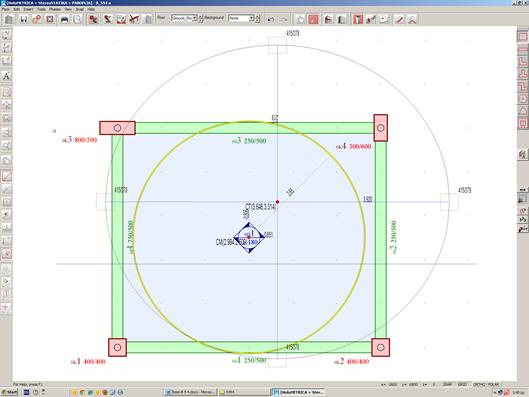The analysis may only be performed using the software. The relevant project is <B_545>. The theory for the analytical determination of the diaphragmatic behaviour at a storey level is described in detail in Appendix D.

The equivalent mass inertial ring is the same as in the two previous methods, since it depends only on the loadings. On the contrary all other quantities are different, as expected. The centre of stiffness CT coordinates are (3.646, 3.314), whereas the torsional stiffness radii are equal to

rx=3.920 m

και

ry=3.572 m

3.910

and

3.080

respectively, assuming fixed-ended columns). The cross-section of the equivalent columns is 415/378 (instead of 524/406).

## Calculation of the diaphragmatic behaviour 1st (and unique) floor level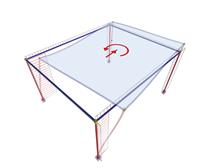Figure 5.4.5.4-2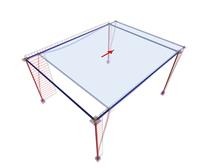Figure 5.4.5.4-3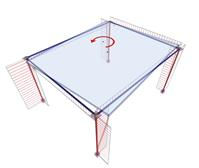Figure 5.4.5.4-4 1st Loading: HX=90.6 kN eccentricity cY=1.0 m MCM,X=90.6 kNm 2nd Loading: HX=90.6 kN Diaphragm restrained against rotation (1st Loading)minus(2nd Loading): HX=0 MCT,X=90.6·yCM+90.6·cY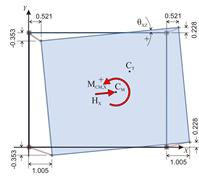Figure 5.4.5.4-5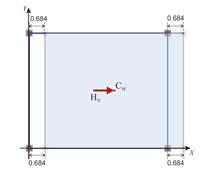Figure 5.4.5.4-6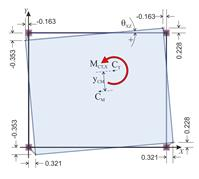Figure 5.4.5.4-7 The displacements of each point i δX,i , δY,i and the rotation angle of the diaphragm θXZ=9.681·10-5 The diaphragm develops zero rotation and moves parallellyto the axes X, Y . Each point of the diaphragm (therefore the CT as well ) has the same principal displacements δXXo=0.684 mm, δXYo=0. The diaphragm develops only a rotation θXZ about CT. The displacements of each point i due to rotation are equal to: δXt,i=δX,i-δXXo, δYt,i=δY,i-δXYo . The CT derives from the expressions: XCT=X1-δYt,1/θXZ=3.646 m YCT =Y1+δXt,1/θXZ=3.316 m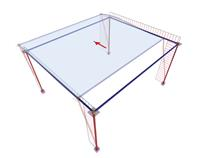HY=90.6 kN

Diaphragm restrained
against rotaion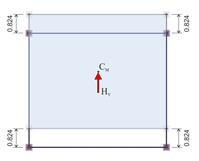Analysis results:

The diaphragm is not rotated, but only translated in parallel to the axes X, Y.

Each point of the diaphragm (therefore and the CT) has the same principal displacement:

δYXo=0, δYYo=0.824 mm.

The 3 rd analysis completes the necessary series of analyses for the determination of all diaphragm data.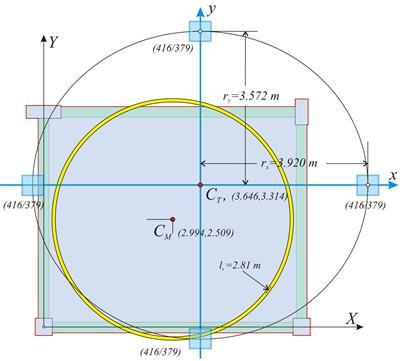Definition of the principal system, of the torsional stiffness radii and of the equivalent system (see §C.6):

tan(2a)=2δXYo/(δXXo-δYYo)=0.0 à 2a=0° à a=0°

δxxo=δXXo=0.684 mm,

δyyo=δYYo=0.824 mm

Kxx=Hx/δxxo=90.6·103m/0.684·10-3m=132.5·106 N/m

Kyy=Hy/δyyo=90.6·103m/0.824·10-3m=110.0·106 N/m

MCT,X=90.6·yCM+90.6·cY=90.6·(3.316-2.500)+90.6·1.0=
=164.5 kNm

Kθ=MCT,X/θXZ=164.5/9.681·10-5=17.0·105kNm

rx=√Kθ/Kyy=√17.0·108N/m/110.0·106N/m=3.931m

ry=√Kθ/Kxx=√17.0·108N/m/132.5·106N/m=3.582 m